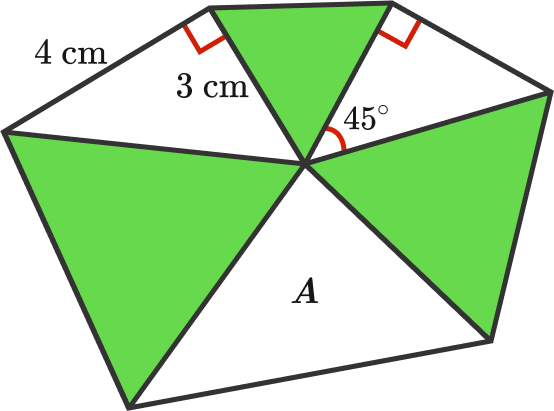# Irregular exagon

Geometry Level 2All the green triangles above are equilateral triangles.

What is the area of the triangle labelled as $A$ $\big($in $\text{cm}^2\big)?$

×